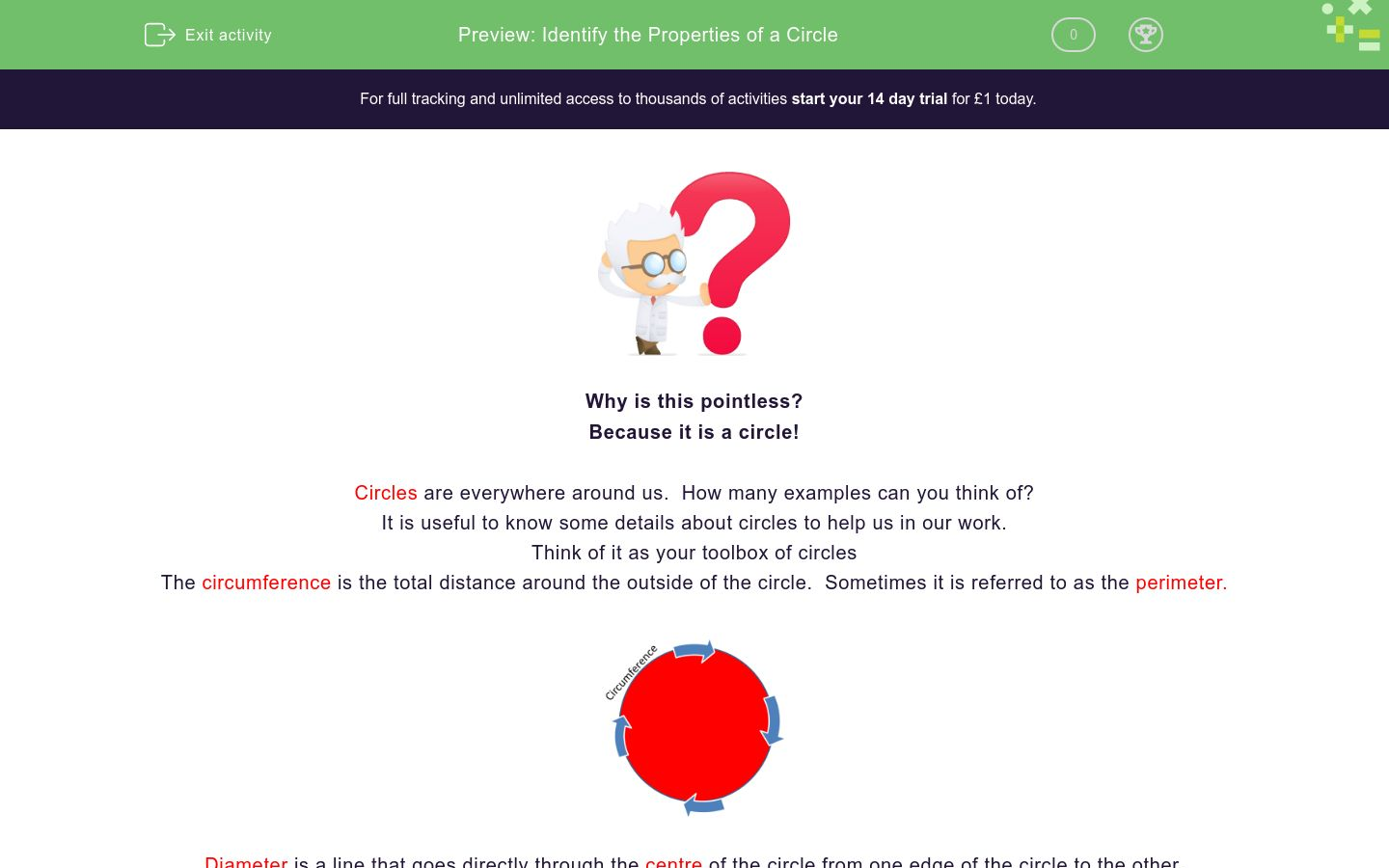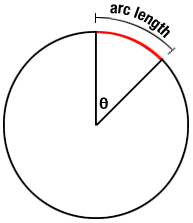# Identify the Properties of a Circle

In this worksheet, students will identify the properties of a circleKey stage:  KS 4

GCSE Subjects:   Maths

GCSE Boards:   OCR, AQA, Eduqas, Pearson Edexcel

Curriculum topic:   Basic Geometry, Geometry and Measures

Curriculum subtopic:   Circles, Properties and Constructions

Difficulty level:### QUESTION 1 of 10Why is this pointless?

Because it is a circle....

Circles are everywhere around us.  How many examples can you think of?

It is useful to know some details about circles to help us in our work.

Think of it as your tool box of circles

Circumference is the total distance around the outside of the circle.  Sometimes it is referred to as the perimeter.Diameter is a line that goes  directly through the centre of the circle from one edge of the circle to the other.Radius is a line that comes from the centre of the circle to the edge.Top Tip

If you have been given the radius and want the diameter,  just double the radius

If you have been given the diameter and want to find the radius, just halve the diameter

A chord is a line that goes from one edge of the circle to another without going through the centre.

There are three chords in this exampleWhen a chord is drawn in a circle, it is split into segments.

The smaller part being the minor segment and the larger part the major segment

A tangent hits the edge of a circle at right angles to the radiusWhat do you call a man who has been in the sun for to long?

A tanned gent.I bet your teacher has never said that one before?Sometimes circles are cut into sectors, like this pizza.  The slice is the minor sector and the rest is the major sector.

An arc - not to be mixed up with the one Noah has, is the part of the circle that forms the outside of the sector.When working with circles we associate two values.

360° for the degrees in a circle(pi) 3.142  You will use these in problem solving in other modules.

Unscramble the following to identify key words associated with a circle.

Start each word with a capital letter.

What is the definition of the circumference of a circle

The distance across a circle

The distance around half of a circle

The total distance around a circle

The total of the part inside the circle

Complete the following

 a line that is drawn through the circle without going through the centre a line that is drawn through the circle and goes through the centre the line that hits the outside of the circle The diameter of a circle is

Complete the following

 a line that is drawn through the circle without going through the centre a line that is drawn through the circle and goes through the centre the line that hits the outside of the circle The diameter of a circle isWhat are the red lines that hit the outside of the circle called.   Start your answer with a capital letter.What is the blue line called?

diameter

chord

arc

Which of the following statements are correct?A tangent is always at right angles to the radius

The radius is perpendicular to the tangent

Where the tangent and the radius meet the angle is 90°

This circle has been divided into 2 sectors

Match the following

## Column B

A chord
does not go through the centre of the circle
A chord
360°
An Arc
Is the outside part of a circle that forms a secto...
Degrees in a circle
cuts the circle into segmentsQuite often diagrams are labelled with letters as shown in this diagram.

What is special about the line  OD and OR?

They are both the same length

One is the diameter the other is the radius

One is a chord the other is the diameterCan you unscramble this sentence to make a statement about a circle?

Ted is red dan char tax grey seed rein rut chee ideal hen

Start your sentence with a capital letter.

• Question 1

Unscramble the following to identify key words associated with a circle.

Start each word with a capital letter.

EDDIE SAYS
Easy peasy for someone like you. Once you know the words associated with a circle your work in this area becomes easier. Why doesn't anyone talk to circles? Because there is no point.
• Question 2

What is the definition of the circumference of a circle

The total distance around a circle
EDDIE SAYS
Try and think of any easy way to remember definitions. The word circumference starts with circ which starts off like a circle, which means it is round. So around the outside springs to mind.
• Question 3

Complete the following

 a line that is drawn through the circle without going through the centre a line that is drawn through the circle and goes through the centre the line that hits the outside of the circle The diameter of a circle is
EDDIE SAYS
It is just one of those things you have to learn. You may be asked to explain how you arrived at an answer so these definitions are important.
• Question 4

Complete the following

EDDIE SAYS
This is really useful to remember particularly when working with circumference and area of a circle. Those sneaky examiners want you to work with the diameter but give you the radius instead.
• Question 5What are the red lines that hit the outside of the circle called.   Start your answer with a capital letter.

Tangents
EDDIE SAYS
If you remember that silly joke in the introduction, an easy way to remember this is the line is outside the circle, like the man was outside in the sun.
• Question 6What is the blue line called?

EDDIE SAYS
I always think of this as something radiating out towards the edge of the circle, that is how I remember radius. Maybe you can think of ways to help you remind you of things. If they are better than mine..let me know.
• Question 7

Which of the following statements are correct?A tangent is always at right angles to the radius
The radius is perpendicular to the tangent
Where the tangent and the radius meet the angle is 90°
This circle has been divided into 2 sectors
EDDIE SAYS
This is just a case of checking you have looked at all the statements and compared them with the answers and dug into your tool box of knowledge. Also there are often different ways of explaining the same thing. Perpendicular means at right angles. There are 90° in a right angle
• Question 8

Match the following

## Column B

A chord
does not go through the centre of...
A chord
cuts the circle into segments
An Arc
Is the outside part of a circle t...
Degrees in a circle
360°
EDDIE SAYS
Dig into your tool box to ensure you are sound on your explanations. These are the added extras in exam marks if you can explain thing clearly.
• Question 9Quite often diagrams are labelled with letters as shown in this diagram.

What is special about the line  OD and OR?

They are both the same length
EDDIE SAYS
Study the diagram carefully. Go from O to D. This is from the centre out to the edge of the circle. Go from O to R. This is also from the centre out to the edge of the circle. They are both a radius and as it doesn't matter which direction from the centre of the circle you go out, the length will always be the same.
• Question 10Can you unscramble this sentence to make a statement about a circle?

Ted is red dan char tax grey seed rein rut chee ideal hen

Start your sentence with a capital letter.

There are three hundred and sixty degrees in a circle
EDDIE SAYS
Once you have got this, then you hopefully won't have to work it out again. I do like a good puzzle though. This is a useful number to have in your tool kit when working with circles as we use it a lot. 360°
---- OR ----

Sign up for a £1 trial so you can track and measure your child's progress on this activity.

### What is EdPlace?

We're your National Curriculum aligned online education content provider helping each child succeed in English, maths and science from year 1 to GCSE. With an EdPlace account you’ll be able to track and measure progress, helping each child achieve their best. We build confidence and attainment by personalising each child’s learning at a level that suits them.

Get started